### 迁移桌面程序到MS Store（11）——应用SVG图标

• 2019 年 11 月 14 日
• 笔记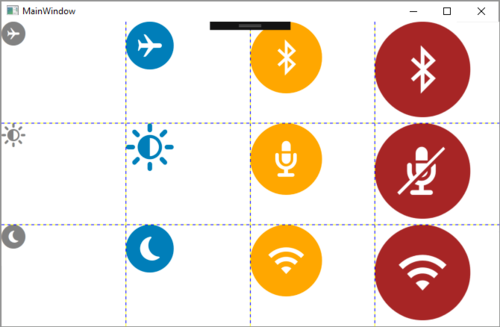从本篇的标题可以看出，我们希望应用SVG矢量图来适应各种分辨率的情况。以WPF程序为例，首先要面对的问题是，WPF并不支持像嵌入JPG/PNG图标这样，直接使用SVG图标。大动干戈的引用第三方library通过自定义类型来支持SVG并不是本文的目的。这里我们要介绍如何通过字体文件，进而在WPF或UWP中使用SVG图标的方式。

https://en.wikipedia.org/wiki/TrueType

https://github.com/manupstairs/WpfAppForFontIcon

`        <Image Grid.Row="0" Grid.Column="0"  Width="32" Height="32" Source="Resources/Airplane_Off.png" ></Image>          <Image Grid.Row="0" Grid.Column="1"  Width="64" Height="64" Source="Resources/Airplane_On.png" ></Image>          <Image Grid.Row="0" Grid.Column="2"  Width="96" Height="96" Source="Resources/Bluetooth_Off.png"  ></Image>          <Image Grid.Row="0" Grid.Column="3"  Width="128" Height="128" Source="Resources/Bluetooth_On.png"  ></Image>`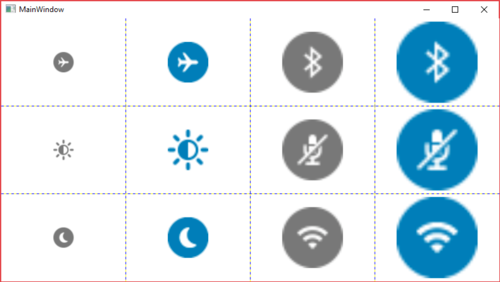`        <TextBlock Grid.Row="0" Grid.Column="0" Text="{x:Static local:FontIcons.airplane_mode_circ}"   Foreground="Gray"  FontSize="32" ></TextBlock>          <TextBlock Grid.Row="0" Grid.Column="1" Text="{x:Static local:FontIcons.airplane_mode_circ}"  Foreground="{StaticResource dellBlue}"  FontSize="64" ></TextBlock>          <TextBlock Grid.Row="0" Grid.Column="2" Text="{x:Static local:FontIcons.bluetooth_inactive}"   Foreground="Orange"  FontSize="96" ></TextBlock>          <TextBlock Grid.Row="0" Grid.Column="3" Text="{x:Static local:FontIcons.bluetooth_inactive}"    Foreground="Brown"  FontSize="128" ></TextBlock>`

https://icomoon.io/app/#/select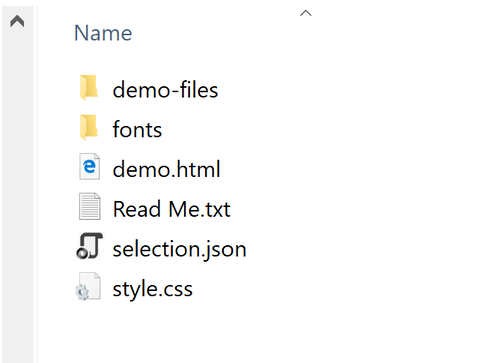ttf文件在fonts文件夹中，实际使用时，需要作为资源文件，添加到WPF工程中。点击图中的demo.html会打开一个本地网页，可用于查找ttf文件中包含的SVG图标，以及对应的unicode。实际我们是通过对unicode的引用来显示SVG图标的。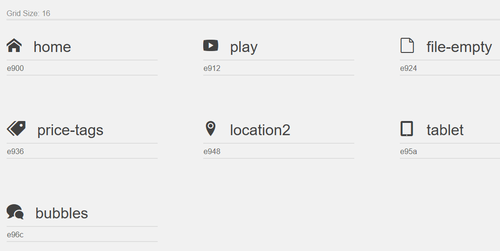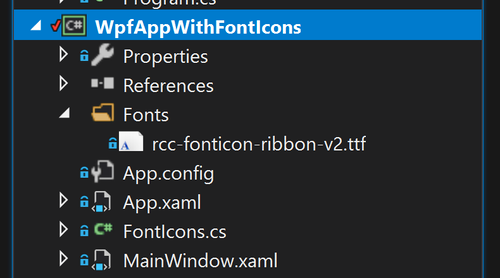ttf字体文件需要以<FontFamily/>的形式添加到项目的<Resources/>节点中。然后再通过<Style/>指定给<TextBlock/>。当然不在<Resources/>节点定义Style，而是在每个<TextBlock/>中指定FontFamily属性也是可以的。有关XAML的语法细节，回字的四种写法什么的，这里略过不提。

`    <Window.Resources>          <FontFamily x:Key="Fonticon">/Fonts/rcc-fonticon-ribbon-v2.ttf#rcc-fonticon-ribbon-v2</FontFamily>          <Style TargetType="TextBlock">              <Setter Property="FontFamily" Value="{StaticResource Fonticon}" ></Setter>          </Style>          <SolidColorBrush x:Key="dellBlue">#007DB8</SolidColorBrush>      </Window.Resources>`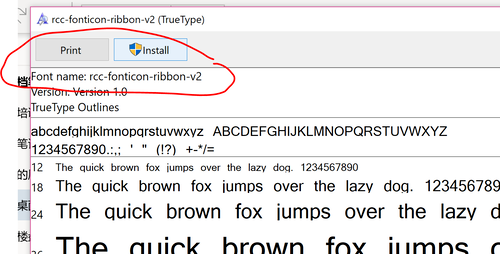`<TextBlock Grid.Row="0" Grid.Column="0" Text="&#xe900;" Foreground="Gray"  FontSize="32" ></TextBlock>`

`textBlockAirplane.Text = "ue900"；`

`    public static class FontIcons      {          public static string airplane_mode_circ { get; } = "ue900";          public static string bluetooth_inactive { get; } = "ue901";          public static string brightness { get; } = "ue902";          public static string brightness_inactive { get; } = "ue903";          public static string browse_inactive { get; } = "ue904";          public static string camera { get; } = "ue905";      }`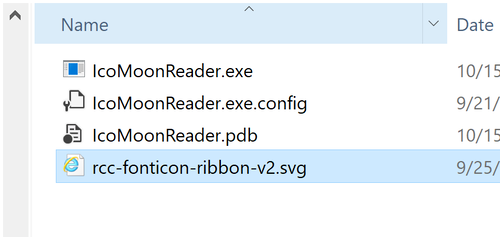其实只有一个方法啦，使用时需要注意具体的文件名是否正确。

`            using (var stream = new FileStream("rcc-fonticon-ribbon-v2.svg", FileMode.Open))              {                  using (var reader = new StreamReader(stream))                  {                      var pattern = "unicode(\S)*\sglyph-name(\S)*"";                      var input = reader.ReadToEnd();                      foreach (Match match in Regex.Matches(input, pattern))                      {                          pattern = ""\S*"";                          var list = new List<string>();                          foreach (var result in Regex.Matches(match.Value, pattern))                          {                              list.Insert(0, result.ToString());                          }                          var name = list.Replace(""", "").Replace("-","_");                          var code = list.Replace("&#x", "\u").Replace(";", "");                          Console.WriteLine(\$"public static string {name} {{ get; }} = {code};");                      }                  }              }`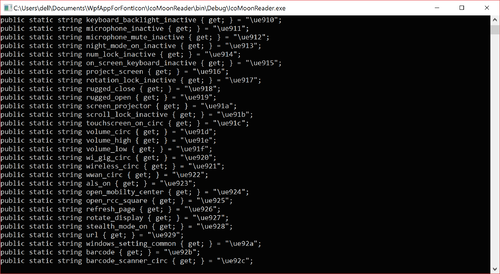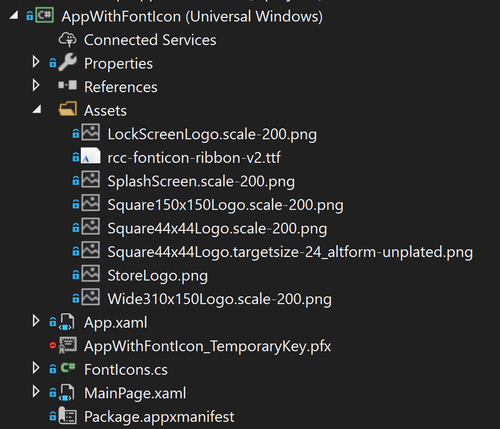`        <TextBlock Grid.Row="0" Grid.Column="0" Text="{x:Bind local:FontIcons.airplane_mode_circ}"   Foreground="Gray"  FontSize="32" ></TextBlock>          <TextBlock Grid.Row="0" Grid.Column="1" Text="{x:Bind local:FontIcons.airplane_mode_circ}"  Foreground="{StaticResource dellBlue}"  FontSize="64" ></TextBlock>          <TextBlock Grid.Row="0" Grid.Column="2" Text="{x:Bind local:FontIcons.bluetooth_inactive}"   Foreground="Orange"  FontSize="96" ></TextBlock>          <TextBlock Grid.Row="0" Grid.Column="3" Text="{x:Bind local:FontIcons.bluetooth_inactive}"    Foreground="Brown"  FontSize="{x:Bind DynamicFontSize(),Mode=OneWay,FallbackValue=128}" ></TextBlock>`

https://github.com/manupstairs/WpfAppForFontIcon